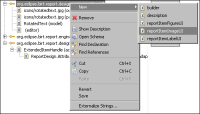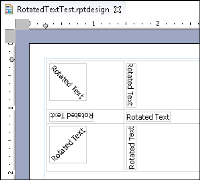Improving the report item UI design
As you see in the report layout in Figure 23‑24, all RotatedText report items in the report design look the same, displayed as horizontal text. Look at the Properties view to see the different angles at which the text would be displayed in the preview. This inconsistency prevents the report developer from getting an intuitive picture of the final report at the design phase. One obvious improvement is to make the report item image appear in the design in the same way in which it appears in the preview. This technique is called WYSIWYG, the familiar acronym for what you see is what you get, that is adopted by most contemporary editors.
To comply with the WYSIWYG paradigm, change the report item presentation to display the rotated text in the layout. Use the Image UI provider or the Figure UI provider instead of the Label UI provider to implement this change.
Image UI provider
The Image UI provider requires an image for the UI presentation. The implementation renders the rotated text as an image and returns it to the caller.
To register the Image UI provider, remove the original Label UI provider first, and then add the new Image UI provider extension, as shown in Figure 23‑26.Figure 23‑26 Changing the UI provider
The implementer class code is shown in Listing 23‑14.
Listing 23‑14 RotatedTextImageUI class
public class RotatedTextImageUI
implements IReportItemImageProvider
{
public void disposeImage( ExtendedItemHandle handle,
Image image )
{
if ( image != null && !image.isDisposed( ) )
{
image.dispose( );
}
}

public Image getImage( ExtendedItemHandle handle )
{
try {
IReportItem item = handle.getReportItem( );

if ( item instanceof RotatedTextItem )
{
int angle =
( (RotatedTextItem) item ).getRotationAngle( );
String text = ( (RotatedTextItem) item ).getText( );

return SwtGraphicsUtil.createRotatedTextImage( text,
angle, null );
}
}
catch ( ExtendedElementException e ) {
e.printStackTrace( );
}
return null;
}
}
The image handler uses Eclipse Standard Widget Toolkit (SWT) to process and rotate the image instead of Swing. An updated version of the SwingGraphicsUtil class is shown in Listing 23‑15.
Listing 23‑15 SwtGraphicsUtil class
public class SwtGraphicsUtil
{
public static Image createRotatedTextImage( String text,
int angle, Font ft )
{
GC gc = null;
try {
if ( text == null || text.trim( ).length( ) == 0 )
{
return null;
}

Display display = Display.getCurrent( );

gc = new GC( display );
if ( ft != null )
{
gc.setFont( ft );
}

Point pt = gc.textExtent( text );

gc.dispose( );

TextLayout tl = new TextLayout( display );
if ( ft != null )
{
tl.setFont( ft );
}
tl.setText( text );

return createRotatedImage( tl, pt.x, pt.y, angle );
}
catch ( Exception e ) {
e.printStackTrace( );

if ( gc != null && !gc.isDisposed( ) )
{
gc.dispose( );
}
}
return null;
}

/**
* @return Returns as [rotatedWidth, rotatedHeight, xOffset,
yOffset]
*/
public static double[] computedRotatedInfo( int width,
int height, int angle )
{
angle = angle % 360;

if ( angle < 0 )
{
angle += 360;
}

if ( angle == 0 )
{
return new double[]{ width, height, 0, 0 };
}
else if ( angle == 90 )
{
return new double[]{ height, width, -width, 0 };
}
else if ( angle == 180 )
{
return new double[]{ width, height, -width, -height };
}
else if ( angle == 270 )
{
return new double[]{ height, width, 0, -height };
}
else if ( angle > 0 && angle < 90 )
{
double angleInRadians = ( ( -angle * Math.PI ) / 180.0 );
double cosTheta = Math.abs( Math.cos( angleInRadians ) );
double sineTheta = Math.abs( Math.sin( angleInRadians ));

int dW = (int) ( width * cosTheta + height * sineTheta );
int dH = (int) ( width * sineTheta + height * cosTheta );

return new double[]{ dW, dH,
-width * sineTheta * sineTheta,
width * sineTheta * cosTheta };
}
else if ( angle > 90 && angle < 180 )
{
double angleInRadians = ( ( -angle * Math.PI ) / 180.0 );
double cosTheta = Math.abs( Math.cos( angleInRadians ) );
double sineTheta = Math.abs( Math.sin( angleInRadians ));

int dW = (int) ( width * cosTheta + height * sineTheta );
int dH = (int) ( width * sineTheta + height * cosTheta );

return new double[]{ dW, dH,
-( width + height * sineTheta * cosTheta ),
-height / 2 };
}
else if ( angle > 180 && angle < 270 )
{
double angleInRadians = ( ( -angle * Math.PI ) / 180.0 );
double cosTheta = Math.abs( Math.cos( angleInRadians ) );
double sineTheta = Math.abs( Math.sin( angleInRadians ));

int dW = (int) ( width * cosTheta + height * sineTheta );
int dH = (int) ( width * sineTheta + height * cosTheta );

return new double[]{ dW, dH,
-( width * cosTheta * cosTheta ),
-( height + width * cosTheta * sineTheta ) };
}
else if ( angle > 270 && angle < 360 )
{
double angleInRadians = ( ( -angle * Math.PI ) / 180.0 );
double cosTheta = Math.abs( Math.cos( angleInRadians ) );
double sineTheta = Math.abs( Math.sin( angleInRadians ));

int dW = (int) ( width * cosTheta + height * sineTheta );
int dH = (int) ( width * sineTheta + height * cosTheta );

return new double[]{ dW, dH,
( height * cosTheta * sineTheta ),
-( height * sineTheta * sineTheta ) };
}

return new double[]{ width, height, 0, 0 };
}

private static Image createRotatedImage( Object src, int width, int height, int angle )
{
angle = angle % 360;

if ( angle < 0 )
{
angle += 360;
}

double[] info = computedRotatedInfo( width, height, angle );

return renderRotatedObject( src, -angle, (int) info,
(int) info, info, info );
}

private static Image renderRotatedObject( Object src,
double angle, int width, int height, double tx, double ty )
{
Display display = Display.getCurrent( );

Image dest = null;
GC gc = null;
Transform tf = null;

try
{
dest = new Image( Display.getCurrent( ), width, height );
gc = new GC( dest );

gc.setAntialias( SWT.ON );
gc.setTextAntialias( SWT.ON );

tf = new Transform( display );
tf.rotate( (float) angle );
tf.translate( (float) tx, (float) ty );

gc.setTransform( tf );

if ( src instanceof TextLayout )
{
TextLayout tl = (TextLayout) src;
tl.draw( gc, 0, 0 );
}
else if ( src instanceof Image )
{
gc.drawImage( (Image) src, 0, 0 );
}
}
catch ( Exception e )
{
e.printStackTrace( );
}
finally
{
if ( gc != null && !gc.isDisposed( ) )
{
gc.dispose( );
}

if ( tf != null && !tf.isDisposed( ) )
{
tf.dispose( );
}
}
return dest;
}
}
Ensure that your RotatedTextImageUI class uses the new SwtGraphicsUtil class and that your ReportItemImageUI item points to the fully qualified RotatedTextImageUI class.
Run another test or run the report design with a grid. On the Layout view, the rotated text items now reflect the rotationAngle setting, as shown in Figure 23‑27. Try changing the Rotation Angle property to see the effect.Figure 23‑27 Report layout of RotatedText implementing Image UI
Preview the report to see the item rendered in an HTML context.
Figure UI provider
The Image UI provides the basic functionality for implementing WYSIWYG support for the rotated report item.
The Figure UI provider is typically used in cases where the requirements are more complex. The Figure UI leverages the IFigure interface from Graphical Editing Framework (GEF) and provides more flexibility and interactivity than the Image UI. Table 23‑10 shows a comparison between Figure UI and Image UI.
 Property Figure UI Image UI Interactivity Supports listeners for UI events. No interactivity support. Life Cycle Three phases:CreateUpdateDisposeCreates the Figure object once. Each time any model state changes, the Update action is invoked. This mechanism supports incremental and selective updates. When Update occurs, the figure instance can check the internal model state and decide what update to execute, full, partial, or none. Two phases:CreateDisposeEach time any model state changes, the Image UI recreates the image presentation. Presentation Supports the creation of hierarchical UI structures, controlled by the GEF layout mechanism. Uses one single Image for presentation. Usage Requires knowledge of GEF framework. Easy to use.
A good use case for the Figure UI provider is one where a mouse middle‑button click controls the report item rotation angle. Each middle‑button click adds 45 degrees to the value of the rotation angle.
To implement the Figure UI provider, first remove any existing Label UI or Image UI provider in the extension points. Then, add the new Figure UI
provider extension, and specify the implementer class as org.eclipse.birt
.sample.reportitem.rotatedtext.RotatedTextFigureUI. The code for the class is in Listing 23‑16.
Listing 23‑16 RotatedTextFigureUI class
public class RotatedTextFigureUI extends ReportItemFigureProvider
{
public IFigure createFigure( ExtendedItemHandle handle )
{
try {
IReportItem item = handle.getReportItem( );

if ( item instanceof RotatedTextItem )
{
return new RotatedTextFigure( (RotatedTextItem) item );
}
}
catch ( ExtendedElementException e ) {
e.printStackTrace( );
}
return null;
}

public void updateFigure( ExtendedItemHandle handle,
IFigure figure )
{
try {
IReportItem item = handle.getReportItem( );

if ( item instanceof RotatedTextItem )
{
RotatedTextFigure fig = (RotatedTextFigure) figure;

fig.setRotatedTextItem( (RotatedTextItem) item );
}
}
catch ( ExtendedElementException e ) {
e.printStackTrace( );
}
}

public void disposeFigure( ExtendedItemHandle handle,
IFigure figure )
{
( (RotatedTextFigure) figure ).dispose( );
}
}
The Figure UI provider creates a RotatedTextFigure instance. The RotatedTextFigure class implements the rendering following an algorithm similar to the Image UI provider. The only addition is using a listener to handle the mouse middle-button click event. The code for this class is in Listing 23‑17.
Listing 23‑17 RotatedTextFigure class
public class RotatedTextFigure extends Figure
{
private String lastText;
private int lastAngle;
private Image cachedImage;
private RotatedTextItem textItem;

RotatedTextFigure( RotatedTextItem textItem )
{
super( );

this.textItem = textItem;

public void mousePressed( MouseEvent me )
{
if ( me.button == 2 )
{
try {
RotatedTextFigure.this.textItem.setRotationAngle(
normalize(
RotatedTextFigure.this.textItem.getRotationAngle( ) + 45 ) );
}
catch ( SemanticException e ) {
e.printStackTrace( );
}
}
}
} );
}

private int normalize( int angle )
{
angle = angle % 360;
if ( angle < 0 )
{
angle += 360;
}
return angle;
}

public Dimension getMinimumSize( int hint, int hint2 )
{
return getPreferredSize( hint, hint2 );
}

public Dimension getPreferredSize( int hint, int hint2 )
{
Display display = Display.getCurrent( );
GC gc = null;

try {
String text = textItem.getText( );
int angle = textItem.getRotationAngle( );

gc = new GC( display );
Point pt = gc.textExtent( text == null ? "" : text );
//\$NON-NLS-1\$
double[] info = SwtGraphicsUtil.computedRotatedInfo(
pt.x, pt.y, angle );

if ( getBorder( ) != null )
{
Insets bdInsets = getBorder( ).getInsets( this );

return new Dimension(
(int) info + bdInsets.getWidth( ),
(int) info + bdInsets.getHeight( ) );
}
return new Dimension( (int) info, (int) info );
}
finally {
if ( gc != null && !gc.isDisposed( ) )
{
gc.dispose( );
}
}
}

protected void paintClientArea( Graphics graphics )
{
final Rectangle r = getClientArea( ).getCopy( );
String text = textItem.getText( );
int angle = textItem.getRotationAngle( );

if ( text == null )
{
text = ""; //\$NON-NLS-1\$
}
if ( !text.equals( lastText ) || angle != lastAngle
|| cachedImage == null || cachedImage.isDisposed( ) )
{
lastText = text;
lastAngle = angle;
if ( cachedImage != null && !cachedImage.isDisposed( ) )
{
cachedImage.dispose( );
}
cachedImage = SwtGraphicsUtil.createRotatedTextImage(
text, angle, null );
}
if ( cachedImage != null && !cachedImage.isDisposed( ) )
{
graphics.drawImage( cachedImage, r.x, r.y );
}
}

void setRotatedTextItem( RotatedTextItem item )
{
this.textItem = item;
}

void dispose( )
{
if ( cachedImage != null && !cachedImage.isDisposed( ) )
{
cachedImage.dispose( );
}
}
}
To test the RotatedText report item, insert a RotatedText item in the layout editor. Each time you middle-click the RotatedText item, the image rotates by 45 degrees.